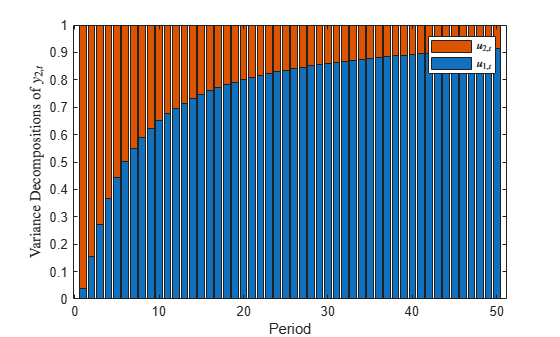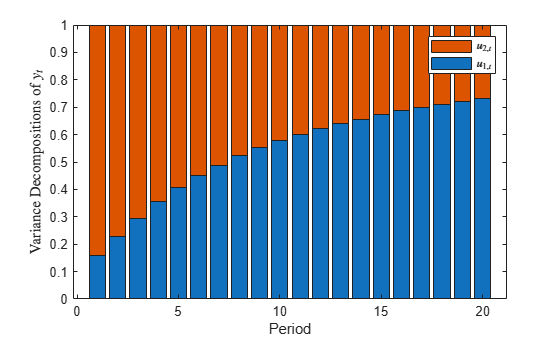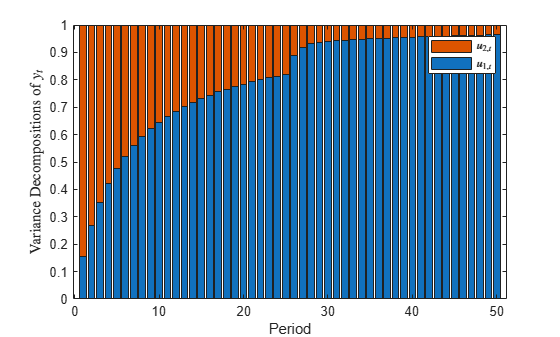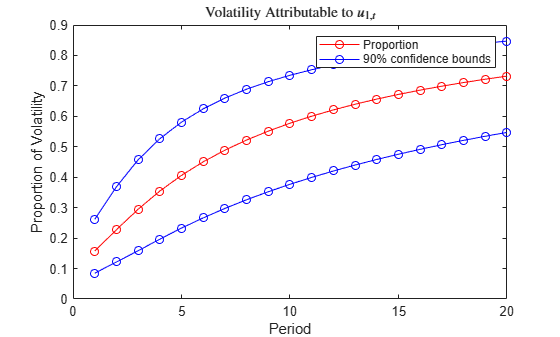# fevd

Generate forecast error variance decomposition (FEVD) of state-space model

## Syntax

``Decomposition = fevd(Mdl)``
``Decomposition = fevd(Mdl,Name,Value)``
``Decomposition = fevd(___,'Params',estParams)``
``[Decomposition,Lower,Upper] = fevd(___,'Params',estParams,'EstParamCov',EstParamCov)``

## Description

The `fevd` function returns the forecast error variance decomposition (FEVD) of the measurement variables in a state-space model attributable to component-wise shocks to each state disturbance. The FEVD provides information about the relative importance of each state disturbance in affecting the forecast error variance of all measurement variables in the system. Other state-space model tools to characterize the dynamics of a specified system include the following:

• The impulse response function (IRF), computed by `irf` and plotted by `irfplot`, traces the effects of a shock to a state disturbance on the state and measurement variables in the system.

• Model-implied temporal correlations, computed by `corr` for a standard state-space model, measure the association between current and lagged state or measurement variables, as prescribed by the form of the model.

### Fully Specified State-Space Model

example

``Decomposition = fevd(Mdl)` returns the FEVD of each measurement variable `Decomposition` of the fully specified state-space model `Mdl`.`

example

``Decomposition = fevd(Mdl,Name,Value)` uses additional options specified by one or more name-value arguments. For example, `'NumPeriods',10` specifies estimating the FEVD for periods 1 through 10.`

### Partially Specified State-Space Model and Confidence Interval Estimation

example

``Decomposition = fevd(___,'Params',estParams)` returns the FEVD of all measurement variables of the partially specified state-space model `Mdl`. `estParams` specifies estimates of all unknown parameters in the model, using any of the input argument combinations in the previous syntaxes.`

example

``[Decomposition,Lower,Upper] = fevd(___,'Params',estParams,'EstParamCov',EstParamCov)` also returns the lower and upper 95% Monte Carlo confidence bounds `Lower` and `Upper` of each measurement variable FEVD. `EstParamCov` specifies the estimated covariance matrix of the parameter estimates, as returned by the `estimate` function, and is required for confidence interval estimation.`

## Examples

collapse all

Compute the model-implied FEVD of two state-space models: one with measurement error and one without measurement error.

Model Without Measurement Error

Explicitly create the state-space model without measurement error

`$\begin{array}{l}{x}_{1,t}={x}_{1,t-1}+0.2{u}_{1,t}\\ {x}_{2,t}={x}_{1,t-1}+0.3{x}_{2,t-1}+{u}_{2,t}\\ {y}_{1,t}={x}_{1,t}\\ {y}_{2,t}={x}_{1,t}+{x}_{2,t}.\end{array}$`

```A = [1 0; 1 0.3]; B = [0.2 0; 0 1]; C = [1 0; 1 1]; Mdl1 = ssm(A,B,C,'StateType',[2 2])```
```Mdl1 = State-space model type: ssm State vector length: 2 Observation vector length: 2 State disturbance vector length: 2 Observation innovation vector length: 0 Sample size supported by model: Unlimited State variables: x1, x2,... State disturbances: u1, u2,... Observation series: y1, y2,... Observation innovations: e1, e2,... State equations: x1(t) = x1(t-1) + (0.20)u1(t) x2(t) = x1(t-1) + (0.30)x2(t-1) + u2(t) Observation equations: y1(t) = x1(t) y2(t) = x1(t) + x2(t) Initial state distribution: Initial state means x1 x2 0 0 Initial state covariance matrix x1 x2 x1 1e+07 0 x2 0 1e+07 State types x1 x2 Diffuse Diffuse ```

`Mdl1` is an `ssm` model object. Because all parameters have known values, the object is fully specified.

Compute the 20-period FEVD of the measurement variables.

```Decomposition1 = fevd(Mdl1); size(Decomposition1)```
```ans = 1×3 20 2 2 ```

`Decomposition` is a 20-by-2-by-2 array representing the 20-period FEVD of the two measurement variables. Display `Decomposition(5,1,2)`.

`Decomposition1(5,1,2)`
```ans = 0.4429 ```

In this case, 44.29% of the volatility of ${\mathit{y}}_{2,\mathit{t}}$ is attributed to the shock applied to ${\mathit{u}}_{1,\mathit{t}-5}$.

Plot the FEVD of ${\mathit{y}}_{2,\mathit{t}}$ for each state disturbance.

```bar(Decomposition1(:,:,2),'stacked') xlabel('Period') ylabel('Variance decompositions of \$y_{2,t}\$','Interpreter','latex') legend('\$u_{1,t}\$','\$u_{2,t}\$','Interpreter','latex')```Because the state-space model is free of measurement error ($\mathit{D}$ = 0), the variance decompositions of each period sum to 1. The volatility attributable to ${\mathit{u}}_{1,\mathit{t}}$ increases with each period.

Model with Measurement Error

Explicitly create the state-space model

`$\begin{array}{l}{x}_{1,t}={x}_{1,t-1}+0.2{u}_{1,t}\\ {x}_{2,t}={x}_{1,t-1}+0.3{x}_{2,t-1}+{u}_{2,t}\\ {y}_{1,t}={x}_{1,t}+{\epsilon }_{1,t}\\ {y}_{2,t}={x}_{1,t}+{x}_{2,t}+{\epsilon }_{2,t}.\end{array}$`

```D = eye(2); Mdl2 = ssm(A,B,C,D,'StateType',[2 2])```
```Mdl2 = State-space model type: ssm State vector length: 2 Observation vector length: 2 State disturbance vector length: 2 Observation innovation vector length: 2 Sample size supported by model: Unlimited State variables: x1, x2,... State disturbances: u1, u2,... Observation series: y1, y2,... Observation innovations: e1, e2,... State equations: x1(t) = x1(t-1) + (0.20)u1(t) x2(t) = x1(t-1) + (0.30)x2(t-1) + u2(t) Observation equations: y1(t) = x1(t) + e1(t) y2(t) = x1(t) + x2(t) + e2(t) Initial state distribution: Initial state means x1 x2 0 0 Initial state covariance matrix x1 x2 x1 1e+07 0 x2 0 1e+07 State types x1 x2 Diffuse Diffuse ```

Compute the 20-period FEVD of the measurement variables.

`Decomposition2 = fevd(Mdl2);`

Plot the FEVD of ${\mathit{y}}_{2,\mathit{t}}$ for each state disturbance.

```bar(Decomposition2(:,:,2),'stacked') xlabel('Period') ylabel('Variance decompositions of \$y_{2,t}\$','Interpreter','latex') legend('\$u_{1,t}\$','\$u_{2,t}\$','Interpreter','latex')```Because the model contains measurement error, the variance proportions do not sum to 1 during each period.

Explicitly create the multivariate diffuse state-space model

`$\begin{array}{l}{x}_{1,t}={x}_{1,t-1}+0.2{u}_{1,t}\\ {x}_{2,t}={x}_{1,t-1}+0.3{x}_{2,t-1}+{u}_{2,t}\\ {y}_{1,t}={x}_{1,t}\\ {y}_{2,t}={x}_{1,t}+{x}_{2,t}.\end{array}$`

```A = [1 0; 1 0.3]; B = [0.2 0; 0 1]; C = [1 0; 1 1]; Mdl = dssm(A,B,C,'StateType',[2 2])```
```Mdl = State-space model type: dssm State vector length: 2 Observation vector length: 2 State disturbance vector length: 2 Observation innovation vector length: 0 Sample size supported by model: Unlimited State variables: x1, x2,... State disturbances: u1, u2,... Observation series: y1, y2,... Observation innovations: e1, e2,... State equations: x1(t) = x1(t-1) + (0.20)u1(t) x2(t) = x1(t-1) + (0.30)x2(t-1) + u2(t) Observation equations: y1(t) = x1(t) y2(t) = x1(t) + x2(t) Initial state distribution: Initial state means x1 x2 0 0 Initial state covariance matrix x1 x2 x1 Inf 0 x2 0 Inf State types x1 x2 Diffuse Diffuse ```

`Mdl` is a `dssm` model object.

Compute the 50-period FEVD of the measurement variables.

```Decomposition = fevd(Mdl,'NumPeriods',50); size(Decomposition)```
```ans = 1×3 50 2 2 ```

Plot the FEVD of ${\mathit{y}}_{2,\mathit{t}}$ for each state disturbance.

```bar(Decomposition(:,:,2),'stacked') xlabel('Period') ylabel('Variance decompositions of \$y_{2,t}\$','Interpreter','latex') legend('\$u_{1,t}\$','\$u_{2,t}\$','Interpreter','latex')```The contribution of ${\mathit{u}}_{1,\mathit{t}}$ to the volatility of ${\mathit{y}}_{2,\mathit{t}}$ approaches 90%.

Simulate data from a known model, fit the data to a state-space model, and then estimate the FEVD of the measurement variables.

Consider the time series decomposition ${\mathit{y}}_{\mathit{t}}={\tau }_{\mathit{t}}+{\mathit{c}}_{\mathit{t}}$, where ${\tau }_{\mathit{t}}$ is a random walk with drift representing the trend component, and ${\mathit{c}}_{\mathit{t}}$ is an AR(1) model representing the cycle component.

`$\begin{array}{l}{\tau }_{t}=3+{\tau }_{t-1}+{u}_{1,t}\\ {c}_{t}=0.5{c}_{t-1}+2{u}_{2,t}.\end{array}$`

The model in state-space notation is

`$\begin{array}{l}{x}_{t}=\left[\begin{array}{c}{\tau }_{t}\\ {d}_{t}\\ {c}_{t}\end{array}\right]=\left[\begin{array}{ccc}1& 3& 0\\ 0& 1& 0\\ 0& 0& 0.5\end{array}\right]\left[\begin{array}{c}{\tau }_{t-1}\\ {d}_{t-1}\\ {c}_{t-1}\end{array}\right]+\left[\begin{array}{cc}1& 0\\ 0& 0\\ 0& 2\end{array}\right]\left[\begin{array}{c}{u}_{1,t}\\ {u}_{2,t}\end{array}\right]\\ {y}_{t}=\left[\begin{array}{ccc}1& 0& 1\end{array}\right]{x}_{t},\end{array}$`

where ${\mathit{d}}_{\mathit{t}}$ is a dummy state representing the drift parameter, which is 1 for all $\mathit{t}$.

Simulate 500 observations from the true model.

```rng(1); % For reproducibility ADGP = [1 3 0; 0 1 0; 0 0 0.5]; BDGP = [1 0; 0 0; 0 2]; CDGP = [1 0 1]; DGP = ssm(ADGP,BDGP,CDGP,'StateType',[2 1 0]); y = simulate(DGP,500);```

Assume that the drift constant, disturbance variances, and AR coefficient are unknown. Explicitly create a state-space model template for estimation that represents the model by replacing the unknown parameters in the model with `NaN`.

```A = [1 NaN 0; 0 1 0; 0 0 NaN]; B = [NaN 0; 0 0; 0 NaN]; C = CDGP; Mdl = ssm(A,B,C,'StateType',[2 1 0]);```

Fit the model template to the data. Specify a set of positive, random standard Gaussian starting values for the four model parameters. Return the estimated model and vector of parameter estimates.

`[EstMdl,estParams] = estimate(Mdl,y,abs(randn(4,1)),'Display','off')`
```EstMdl = State-space model type: ssm State vector length: 3 Observation vector length: 1 State disturbance vector length: 2 Observation innovation vector length: 0 Sample size supported by model: Unlimited State variables: x1, x2,... State disturbances: u1, u2,... Observation series: y1, y2,... Observation innovations: e1, e2,... State equations: x1(t) = x1(t-1) + (2.91)x2(t-1) + (0.92)u1(t) x2(t) = x2(t-1) x3(t) = (0.52)x3(t-1) + (2.13)u2(t) Observation equation: y1(t) = x1(t) + x3(t) Initial state distribution: Initial state means x1 x2 x3 0 1 0 Initial state covariance matrix x1 x2 x3 x1 1.00e+07 0 0 x2 0 0 0 x3 0 0 6.20 State types x1 x2 x3 Diffuse Constant Stationary ```
```estParams = 4×1 2.9115 0.5189 0.9200 2.1278 ```

`EstMdl` is a fully specified `ssm` model object. Model estimates are close to their true values.

Compute and plot the FEVD of the measurement variable. Specify the model template `Mdl` and the vector of estimated parameters `estParams`.

```Decomposition = fevd(Mdl,'Params',estParams); bar(Decomposition,'stacked') xlabel('Period') ylabel('Variance decompositions of \$y_{t}\$','Interpreter','latex') legend('\$u_{1,t}\$','\$u_{2,t}\$','Interpreter','latex')```Noise in the cyclical component dominates the volatility of the measurement variable in low lags, with increasing contribution from the trend component noise as the lag increases.

Simulate data from a time-varying state-space model, fit a model to the data, and then estimate the time-varying FEVD of the measurement variable.

Consider the time series decomposition ${\mathit{y}}_{\mathit{t}}={\tau }_{\mathit{t}}+{\mathit{c}}_{\mathit{t}}$, where ${\tau }_{\mathit{t}}$ is a random walk with drift representing the trend component, and ${\mathit{c}}_{\mathit{t}}$ is an AR(1) model representing the cyclical component. Suppose that the cyclical component changes during period 26 over a 50-period time span.

`$\begin{array}{l}{\tau }_{t}=1+{\tau }_{t-1}+{u}_{1,t}\\ {c}_{t}=\left\{\begin{array}{cc}0.5{c}_{t}+2{u}_{2,t};& t<26\\ -0.2{c}_{t}+0.5{u}_{2,t};& t\ge 26\end{array}.\end{array}$`

The function `timeVariantTrendCycleParamMap.m`, stored in `mlr/examples/econ/main`, specifies the model structure. `mlr` is the value of `matlabroot`.

`type timeVariantTrendCycleParamMap.m`
```% Copyright 2021 The MathWorks, Inc. function [A,B,C,D,Mean0,Cov0,StateType] = timeVariantTrendCycleParamMap(params) % Time-varying state-space model parameter mapping function example. This % function maps the vector params to the state-space matrices (A, B, C, and % D). The measurement equation is a times series decomposed into trend and % cyclical components, with a structural break in the cycle during period % 26. % % The trend component is tau_t = drift + tau_{t-1} + s_1u1_t. % % The cyclical component is: % * c_t = phi_1*c_{t-1} + s_2*u2_t; t = 1 through 25 % * c_t = phi_2*c_{t-1} + s_3*u2_t; t = 11 through 26. % % The measurement equation is y_t = tau_t + c_t. A1 = {[1 params(1) 0; 0 1 0; 0 0 params(2)]}; A2 = {[1 params(1) 0; 0 1 0; 0 0 params(3)]}; varu1 = exp(params(4)); % Positive variance constraints varu21 = exp(params(5)); varu22 = exp(params(6)); B1 = {[sqrt(varu1) 0; 0 0; 0 sqrt(varu21)]}; B2 = {[sqrt(varu1) 0; 0 0; 0 sqrt(varu22)]}; C = [1 0 1]; D = 0; sc = 25; A = [repmat(A1,sc,1); repmat(A2,sc,1)]; B = [repmat(B1,sc,1); repmat(B2,sc,1)]; Mean0 = []; Cov0 = []; StateType = [2 1 0]; end ```

Implicitly create a partially specified state-space model representing the data generating process (DGP).

```ParamMap = @timeVariantTrendCycleParamMap; DGP = ssm(ParamMap);```

Simulate 50 observations from the DGP. Because `DGP` is partially specified, pass the true parameter values to `simulate` by using the `'Params'` name-value argument.

```rng(5) % For reproducibility trueParams = [1 0.5 -0.2 2*log(1) 2*log(2) 2*log(0.5)]; % Transform variances for parameter map y = simulate(DGP,50,'Params',trueParams);```

`y` is a 50-by-1 vector of simulated measurements ${\mathit{y}}_{\mathit{t}}$ from the DGP.

Because `DGP` is a partially specified, implicit model object, its parameters are unknown. Therefore, it can serve as a model template for estimation.

Fit the model to the simulated data. Specify random standard Gaussian draws for the initial parameter values, and turn off the estimation display. Return the parameter estimates.

`[~,estParams] = estimate(DGP,y,randn(1,6),'Display','off')`
```estParams = 1×6 0.8510 0.0118 0.6309 -0.3227 1.3778 -0.2200 ```

`estParams` is a 1-by-6 vector of parameter estimates. The output argument list of the parameter mapping function determines the order of the estimates.

Estimate the FEVD of the measurement variable by supplying `DGP` (not the estimated model) and the estimated parameters using the `'Params'` name-value argument.

```Decomposition = fevd(DGP,'Params',estParams,'NumPeriods',50); bar(Decomposition,'stacked') xlabel('Period') ylabel('Variance decompositions of \$y_{t}\$','Interpreter','latex') legend('\$u_{1,t}\$','\$u_{2,t}\$','Interpreter','latex')```The FEVD jumps at period 26, when the structural break occurs.

Simulate data from a known model, fit the data to a state-space model, and then estimate the FEVD of the measurement variables with 90% Monte Carlo confidence bounds.

Consider the time series decomposition ${\mathit{y}}_{\mathit{t}}={\tau }_{\mathit{t}}+{\mathit{c}}_{\mathit{t}}$, where ${\tau }_{\mathit{t}}$ is a random walk with drift representing the trend component, and ${\mathit{c}}_{\mathit{t}}$ is an AR(1) model representing the cycle component.

`$\begin{array}{l}{\tau }_{t}=3+{\tau }_{t-1}+{u}_{1,t}\\ {c}_{t}=0.5{c}_{t-1}+2{u}_{2,t}.\end{array}$`

The model in state-space notation is

`$\begin{array}{l}{x}_{t}=\left[\begin{array}{c}{\tau }_{t}\\ {d}_{t}\\ {c}_{t}\end{array}\right]=\left[\begin{array}{ccc}1& 3& 0\\ 0& 1& 0\\ 0& 0& 0.5\end{array}\right]\left[\begin{array}{c}{\tau }_{t-1}\\ {d}_{t-1}\\ {c}_{t-1}\end{array}\right]+\left[\begin{array}{cc}1& 0\\ 0& 0\\ 0& 2\end{array}\right]\left[\begin{array}{c}{u}_{1,t}\\ {u}_{2,t}\end{array}\right]\\ {y}_{t}=\left[\begin{array}{ccc}1& 0& 1\end{array}\right]{x}_{t},\end{array}$`

where ${\mathit{d}}_{\mathit{t}}$ is a dummy state representing the drift parameter, which is 1 for all t.

Simulate 500 observations from the true model.

```rng(1); % For reproducibility ADGP = [1 3 0; 0 1 0; 0 0 0.5]; BDGP = [1 0; 0 0; 0 2]; CDGP = [1 0 1]; DGP = ssm(ADGP,BDGP,CDGP,'StateType',[2 1 0]); y = simulate(DGP,500);```

Assume that the drift constant, disturbance variances, and AR coefficient are unknown. Explicitly create a state-space model template for estimation that represents the model by replacing the unknown parameters in the model with `NaN`.

```A = [1 NaN 0; 0 1 0; 0 0 NaN]; B = [NaN 0; 0 0; 0 NaN]; C = CDGP; Mdl = ssm(A,B,C,'StateType',[2 1 0]);```

Fit the model template to the data. Specify a set of positive, random standard Gaussian starting values for the four model parameters, and turn off the estimation display. Return the estimated model and vector of parameter estimates and their estimated covariance matrix.

`[EstMdl,estParams,EstParamCov] = estimate(Mdl,y,abs(randn(4,1)),'Display','off');`

`EstMdl` is a fully specified `ssm` model object. Model estimates are close to their true values.

Compute the FEVD of the measurement variable with period-wise 90% Monte Carlo confidence bounds. Specify the model template `Mdl`, vector of estimated parameters `estParams`, and their estimated covariance matrix `EstParamCov`.

```[Decomposition,Lower,Upper] = fevd(Mdl,'Params',estParams,'EstParamCov',EstParamCov,... 'Confidence',0.9);```

Plot the proportion of volatility of ${\mathit{y}}_{\mathit{t}}$ attributable to ${\mathit{u}}_{1,\mathit{t}}$ with corresponding 90% confidence bounds.

```plot(Decomposition(:,1),'r-o') hold on plot([Lower(:,1) Upper(:,1)],'b-o') hold off xlabel('Period') ylabel('Proportion of volatility') title('Volatility Attributable to \$u_{1,t}\$','Interpreter','latex') legend('Proportion','90% confidence bounds')```The confidence bounds are initially relatively tight, but widen as the lag and volatility increase.

## Input Arguments

collapse all

State-space model, specified as an `ssm` model object returned by `ssm` or its `estimate` function, or a `dssm` model object returned by `dssm` or its `estimate` function.

If `Mdl` is partially specified (that is, it contains unknown parameters), specify estimates of the unknown parameters by using the `'Params'` name-value argument. Otherwise, `fevd` issues an error.

`fevd` issues an error when `Mdl` is a dimension-varying model, which is a time-varying model containing at least one variable that changes dimension during the sampling period (for example, a state variable drops out of the model).

Tip

If `Mdl` is fully specified, you cannot estimate confidence bounds. To estimate confidence bounds:

1. Create a partially specified state-space model template for estimation `Mdl`.

2. Estimate the model by using the `estimate` function and data. Return the estimated parameters `estParams` and estimated parameter covariance matrix `EstParamCov`.

3. Pass the model template for estimation `Mdl` to `fevd`, and specify the parameter estimates and covariance matrix by using the `'Params'` and `'EstParamCov'` name-value arguments.

4. For the `fevd` function, return the appropriate output arguments for lower and upper confidence bounds.

### Name-Value Arguments

Specify optional comma-separated pairs of `Name,Value` arguments. `Name` is the argument name and `Value` is the corresponding value. `Name` must appear inside quotes. You can specify several name and value pair arguments in any order as `Name1,Value1,...,NameN,ValueN`.

Example: `'NumPeriods',10` estimating the FEVD for specifies estimating the FEVD for periods 1 through 10.
FEVD Options

collapse all

Number of periods for which `fevd` computes the FEVD, specified as a positive integer. Periods in the FEVD start at time 1 and end at time `NumPeriods`.

Example: `'NumPeriods',10` specifies the inclusion of 10 consecutive time points in the FEVD, starting at time 1 and ending at time 10.

Data Types: `double`

Estimates of the unknown parameters in the partially specified state-space model `Mdl`, specified as a numeric vector.

If `Mdl` is partially specified (contains unknown parameters specified by `NaN`s), you must specify `Params`. The `estimate` function returns parameter estimates of `Mdl` in the appropriate form. However, you can supply custom estimates by arranging the elements of `Params` as follows:

• If `Mdl` is an explicitly created model (`Mdl.ParamMap` is empty `[]`), arrange the elements of `Params` to correspond to hits of a column-wise search of `NaN`s in the state-space model coefficient matrices, initial state mean vector, and covariance matrix.

• If `Mdl` is time invariant, the order is `A`, `B`, `C`, `D`, `Mean0`, and `Cov0`.

• If `Mdl` is time varying, the order is `A{1}` through `A{end}`, `B{1}` through `B{end}`, `C{1}` through `C{end}`, `D{1}` through `D{end}`, `Mean0`, and `Cov0`.

• If `Mdl` is an implicitly created model (`Mdl.ParamMap` is a function handle), the first input argument of the parameter-to-matrix mapping function determines the order of the elements of `Params`.

If `Mdl` is fully specified, `fevd` ignores `Params`.

Example: Consider the state-space model `Mdl` with `A = B = [NaN 0; 0 NaN]` , `C = [1; 1]`, `D = 0`, and initial state means of 0 with covariance `eye(2)`. `Mdl` is partially specified and explicitly created. Because the model parameters contain a total of four `NaN`s, `Params` must be a 4-by-1 vector, where `Params(1)` is the estimate of `A(1,1)`, `Params(2)` is the estimate of `A(2,2)`, `Params(3)` is the estimate of `B(1,1)`, and `Params(4)` is the estimate of `B(2,2)`.

Data Types: `double`

Confidence Bound Estimation Options

collapse all

Estimated covariance matrix of unknown parameters in the partially specified state-space model `Mdl`, specified as a positive semidefinite numeric matrix.

`estimate` returns the estimated parameter covariance matrix of `Mdl` in the appropriate form. However, you can supply custom estimates by setting `EstParamCov(i,j)` to the estimated covariance of the estimated parameters `Params(i)` and `Params(j)`, regardless of whether `Mdl` is time invariant or time varying.

If `Mdl` is fully specified, `fevd` ignores `EstParamCov`.

By default, `fevd` does not estimate confidence bounds.

Data Types: `double`

Number of Monte Carlo sample paths (trials) to generate to estimate confidence bounds, specified as a positive integer.

Example: `'NumPaths',5000`

Data Types: `double`

Confidence level for the confidence bounds, specified as a numeric scalar in the interval [0,1].

For each period, randomly drawn confidence intervals cover the true response `100*Confidence`% of the time.

The default value is `0.95`, which implies that the confidence bounds represent 95% confidence intervals.

Data Types: `double`

## Output Arguments

collapse all

FEVD of the measurement variables yt, returned as a `NumPeriods`-by-k-by-n numeric array.

`Decomposition(t,i,j)` is the FEVD of measurement variable `j` at period `t`, when a unit shock is applied to state-disturbance variable `i` during period 1, for `t` = 1,2,...,`NumPeriods`, `i` = 1,2,...,k, and `j` = 1,2,...,n.

Pointwise lower confidence bounds of the FEVD of the measurement variables, returned as a `NumPeriods`-by-k-by-n numeric array.

`Lower(t,i,j)` is the lower bound of the `100*Confidence`% percentile interval on the true FEVD of measurement variable `j` at period `t`, when a unit shock is applied to state-disturbance variable `i` during period 1.

Pointwise upper confidence bounds of the FEVD of the measurement variables, returned as a `NumPeriods`-by-k-by-n numeric array.

`Upper(t,i,j)` is the upper confidence bound corresponding to the lower confidence bound `Lower``(t,i,j)`.

collapse all

### Forecast Error Variance Decomposition

The forecast error variance decomposition (FEVD) of a state-space model measures the volatility in each measurement variable yt as a result of a unit impulse to each state disturbance ut at period 1. The FEVD tracks the volatility as the impulses propagate the system for each period t ≥ 1. The FEVD provides information about the relative importance of each state disturbance in affecting the forecast error variance of all measurement variables in the system.

Consider the time-invariant state-space model at time t

`$\begin{array}{l}{x}_{t}=A{x}_{t-1}+B{u}_{t}\\ {y}_{t}=C{x}_{t}+D{\epsilon }_{t},\end{array}$`

and consider unit shocks to all state disturbances ut during period ts, where s < t.

The state equation, expressed as a function of uts is ${x}_{t}={A}^{s+1}{x}_{t-s-1}+\sum _{i=0}^{s}{A}^{i}B{u}_{t-i}.$ The corresponding measurement equation is

`${y}_{t}=C{A}^{s+1}{x}_{t-s-1}+C\sum _{i=0}^{s}{A}^{i}B{u}_{t-i}+D{\epsilon }_{t}.$`

Therefore, the total volatility of yt, attributed to shocks from periods ts through t, is

`${V}_{s}=C\left(\sum _{i=0}^{s}{A}^{i}B{B}^{\prime }{\left({A}^{i}\right)}^{\prime }\right){C}^{\prime }+D{D}^{\prime }.$`

This result implies that noise in both the transition and measurement equations contributes to the forecast error variance.

The volatility attributed to state disturbance j uj,t is

`${V}_{sj}=C\left(\sum _{i=0}^{s}{A}^{i}B{I}_{k}^{\left(j\right)}{B}^{\prime }{\left({A}^{i}\right)}^{\prime }\right){C}^{\prime },$`

where:

• Ik(j) is a k-by-k selection matrix, a matrix of zeros except for the value 1 in element (j,j).

• ${V}_{s}=\sum _{j=1}^{k}{V}_{sj}+D{D}^{\prime }$

As a result, the s-step ahead forecast error variance of yi,t, attributable to a unit shock to uj,t, is

`${\gamma }_{s,ij}=\frac{{V}_{js}\left(i,i\right)}{{V}_{s}\left(i,i\right)}.$`

If D is zero, the FEVD of a measurement variable at period t sums to one (in other words, the sum of each row is one). Otherwise, the FEVD of a measurement variable at period t does not necessarily sum to one; the remaining portion is attributable to DD'.

The FEVD of a time-varying, dimension-invariant state-space model is also time varying. In this case, `fevd` always applies the unit shock during period 1. For an s-period-ahead FEVD, the measurement equation is

`${y}_{s}={C}_{s}{A}_{s}\cdots {A}_{2}{A}_{1}{x}_{0}+{C}_{s}\sum _{i=1}^{s-1}\left(\prod _{\ell =1}^{i}{A}_{s-\ell +1}\right){B}_{s-i}{u}_{s-i}+{C}_{s}{B}_{s}{u}_{s}+{D}_{s}{\epsilon }_{s}.$`

The total volatility of ys is

`${V}_{s}={C}_{s}\left\{\sum _{i=1}^{s-1}\left[\left(\prod _{\ell =1}^{i}{A}_{s-\ell +1}\right){B}_{s-i}{B}_{s-i}^{\prime }{\left(\prod _{\ell =1}^{i}{A}_{s-\ell +1}\right)}^{\prime }\right]\right\}{C}_{s}{}^{\prime }+{D}_{s}{D}_{s}{}^{\prime }.$`

As with time-invariant models, the s-period-ahead volatility attributed to state disturbance j shocked during period 1 uj,1 is

`${V}_{sj}={C}_{s}\left\{\sum _{i=1}^{s-1}\left[\left(\prod _{\ell =1}^{i}{A}_{s-\ell +1}\right){B}_{s-i}{I}_{k}^{\left(j\right)}{B}_{s-i}^{\prime }{\left(\prod _{\ell =1}^{i}{A}_{s-\ell +1}\right)}^{\prime }\right]\right\}{C}_{s}{}^{\prime }.$`

As a result, the s-step-ahead forecast error variance of yi,s, attributable to a unit shock to uj,1, is

`${\gamma }_{s,ij}=\frac{{V}_{js}\left(i,i\right)}{{V}_{s}\left(i,i\right)}.$`

Because time-invariant and time-varying FEVDs do not include initial state distribution terms, the formulas apply to standard and diffuse state-space models.

## Algorithms

• If you do not supply the `EstParamCov` name-value argument, confidence bounds of each period overlap.

• `fevd` uses Monte Carlo simulation to compute confidence intervals.

1. `fevd` randomly draws `NumPaths` variates from the asymptotic sampling distribution of the unknown parameters in `Mdl`, which is Np(`Params`,`EstParamCov`), where p is the number of unknown parameters.

2. For each randomly drawn parameter set j, `fevd` does the following:

1. Create a state-space model that is equal to `Mdl`, but substitute in parameter set j.

2. Compute the random FEVD of the resulting model γj(t), where t = 1 through `NumPaths`.

3. For each time t, the lower bound of the confidence interval is the `(1 – c)/2` quantile of the simulated FEVD at period t γ(t), where `c` = `Confidence`. Similarly, the upper bound of the confidence interval at time t is the `(1 – c)/2` upper quantile of γ(t).

### Topics

Introduced in R2021a